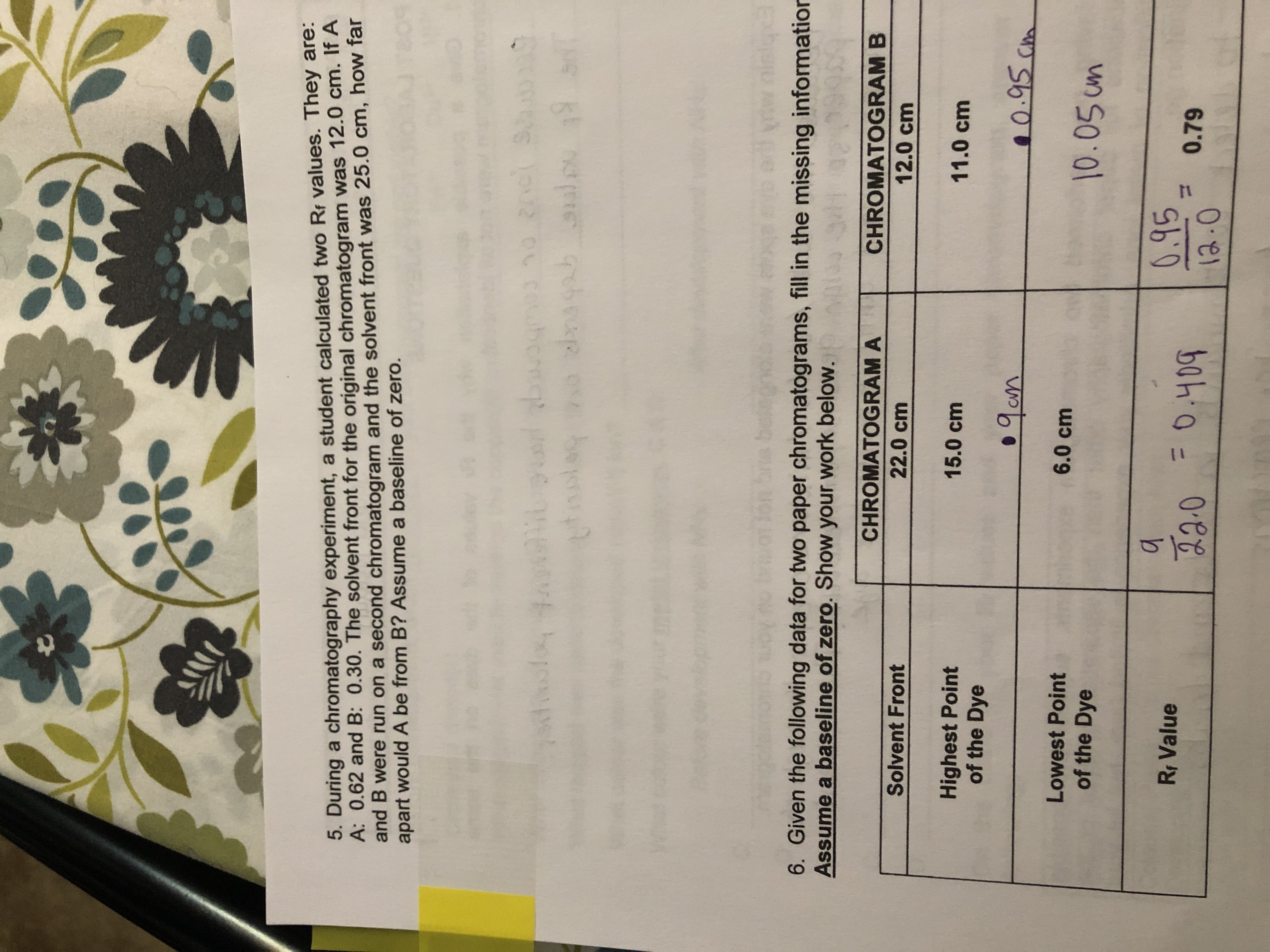# 5. During a chromatography experiment, a student calculated two Rr values. They are: A: 0.62 and B: 0.30. The solvent front for the original chromatogram was 12.0 cm. If A and B were run on a second chromatogram and the solvent front was 25.0 cm, how far apart would A be from B? Assume a baseline of zero. 93 n0 20c 9 19 qsbtup wogh db *444 99 6. Given the following data for two paper chromatograms, fill in the missing information Assume a baseline of zero. Show your work below. 341 CHROMATOGRAM A CHROMATOGRAM B Solvent Front 22.0 cm 12.0 cm Highest Point of the Dye 15.0 cm 11.0 cm 0.95 Ca Lowest Point 6.0 cm 10.05um of the Dye 0.95 Rr Value ం 200.409 0.79 a.0

Question

Question 5help_outlineImage Transcriptionclose5. During a chromatography experiment, a student calculated two Rr values. They are: A: 0.62 and B: 0.30. The solvent front for the original chromatogram was 12.0 cm. If A and B were run on a second chromatogram and the solvent front was 25.0 cm, how far apart would A be from B? Assume a baseline of zero. 93 n0 20c 9 19 qsbtup wogh db *444 99 6. Given the following data for two paper chromatograms, fill in the missing information Assume a baseline of zero. Show your work below. 341 CHROMATOGRAM A CHROMATOGRAM B Solvent Front 22.0 cm 12.0 cm Highest Point of the Dye 15.0 cm 11.0 cm 0.95 Ca Lowest Point 6.0 cm 10.05um of the Dye 0.95 Rr Value ం 200.409 0.79 a.0 fullscreen

### Want to see this answer and more?

Experts are waiting 24/7 to provide step-by-step solutions in as fast as 30 minutes!*